# Non Verbal Reasoning - Cubes and Dice

### Exercise :: Cubes and Dice - Section 1

The sheet of paper shown in the figure (X) given on the left hand side, in each problem, is folded to form a box. Choose from amongst the alternatives (1), (2), (3) and (4), the boxes that are similar to the box that will be formed.

21.

Choose the box that is similar to the box formed from the given sheet of paper (X).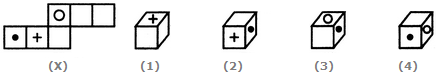A. 1 only B. 1, 2 and 3 only C. 2 and 3 only D. 1, 2, 3 and 4

Explanation:

The fig. (X) is similar to the Form V. So, when the sheet in fig. (X) is folded to form a cube, then the face bearing a dot appears opposite to a blank face, the face bearing a '+' sign appears opposite to another blank face and the face bearing a circle appears opposite to the third blank face. Clearly, all the four cubes shown in figures (1), (2), (3) and (4) can be formed.

22.

Choose the box that is similar to the box formed from the given sheet of paper (X).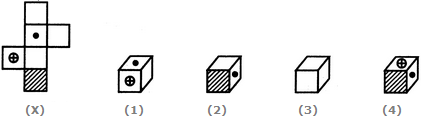A. 1 only B. 1 and 3 only C. 1, 3 and 4 only D. 1, 2, 3 and 4

Explanation:

The fig. (X) is similar to Form IV. So, when the sheet shown in fig. (X) is folded to , form a cube, then the face bearing the dot lies opposite to the shaded face, the face bearing a circle (with '+' sign inside it) lies opposite to a blank face and the remaining two blank faces lie opposite to each other. Clearly, the cubes shown in figures (2) and (4) cannot be formed since they have the shaded face adjacent to the face bearing a dot and the cube shown in fig. (3) cannot be formed since it shows all the three blank faces adjacent to each other. Hence only the cube shown in fig. (1) can be formed.

23.

Choose the box that is similar to the box formed from the given sheet of paper (X).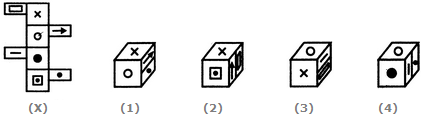A. 1 and 2 only B. 1 and 3 only C. 3 and 4 only D. 1, 2, 3 and 4

The fig. (X) is similar to the Form VIII. So, when a cube is formed by folding the sheet shown in fig. (X), then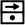and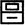are the two faces and these two faces lie opposite to each other. Also, the face bearing the 'x' sigh lies opposite to the face bearing the black circle and the face bearing the white circle lies opposite to the face bearing the square (having a dot inside it). Now, the cubes in figures (2) and (4) consist of faces which are not formed when the sheet in fig. (X) is folded. Hence, these two cubes are not, formed. Therefore, only the cubes in figures (1) and (3) are formed.